# amazon-interview-questionsMove Zeroes

Given an array X[] of n elements filled with several integers, some of them being zeroes, write a program to move all the zeros to the end. We need to preserve the relative order of non-zero elements and solve this problem in place using O(n) time complexity. Note: This is an excellent problem to learn problem solving using two pointers approach.Median of Two Sorted Arrays

There are two sorted arrays A and B of size n each, write a program to find the median of these two sorted arrays obtained after merging (the new merged array will be an array of length 2n). The median of a sorted array of size n is defined as the middle element when n is odd and the average of the middle two elements when n is even.Pair sum in an array

Given an array of n integers and a target number, write a program to find whether a pair sum exists in the array or not. In other words, we need to check for a pair of elements in the array that sum exactly to the target value. Assume that all elements are distinct. Note: This is an excellent problem to learn problem solving using two pointers and hash table.Minimum Number of Jumps to Reach End

An array of non-negative integers is given and the aim is to reach the last index in the minimum number of jumps. You are initially positioned at the first index of the array and each element in the array represents your maximum jump length at that position. Note: This is an excellent problem to learn problem solving using dynamic programming.Given an array of n elements, write a program to find the maximum subarray sum. A subarray of array X[] is a contiguous segment from X[i] to X[j], where 0 <= i <= j <= n-1. Note: Max subarray sum is an excellent problem to learn problem-solving using the divide and conquer approach, dynamic programming, and single loop (kadane's algorithm).Flood Fill Algorithm

The flood fill problem is a way to fill a region of connected pixels with a new colour, starting from a given pixel in an image. You are given an image represented by an m x n grid of integers, where the image[i] represents the pixel value at position (i, j). You are also given the coordinates of a starting pixel (x, y) and a new colour to use for the flood fill operation.Generating all K-combinations

Given two numbers, n and K, write a program to find all possible combinations of K numbers from 1 to n. You may return the answer in any order. Note: This is an excellent problem for learning problem-solving using the inclusion and exclusion principle of combinatorics and backtracking. We can apply similar ideas to solve other problems.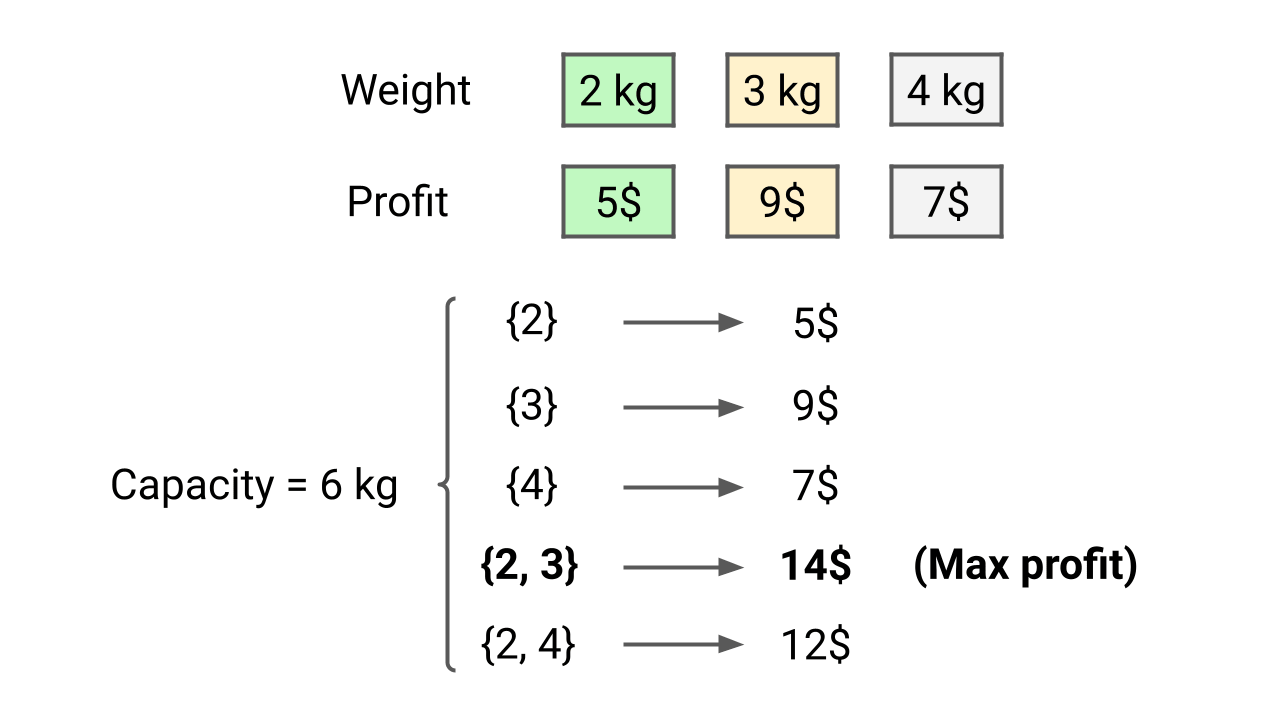0-1 Knapsack Problem

We are given n items, where each item has a weight and a profit associated with it. We are also given a knapsack with a capacity of C (the knapsack can hold a maximum weight of C). Write a program to determine the maximum profit that can be obtained by selecting a subset of items without exceeding the knapsack's capacity.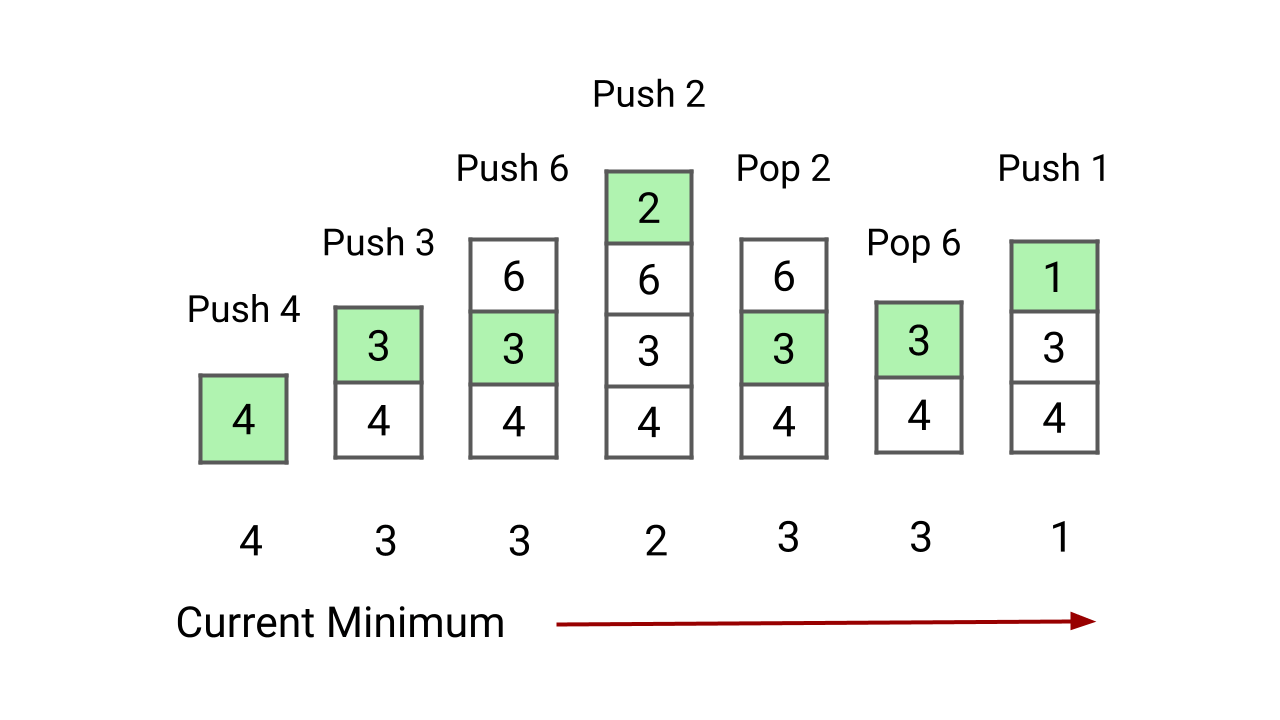Min Stack Problem

Design and implement a stack that supports the push, pop, top, and retrieval of the minimum element in constant time. In other words, our goal is to implement the MinStack class, which supports push, pop, top, and getMin operations with O(1) time complexity. Note: The pop, top, and getMin operations will always be called on non-empty stacks.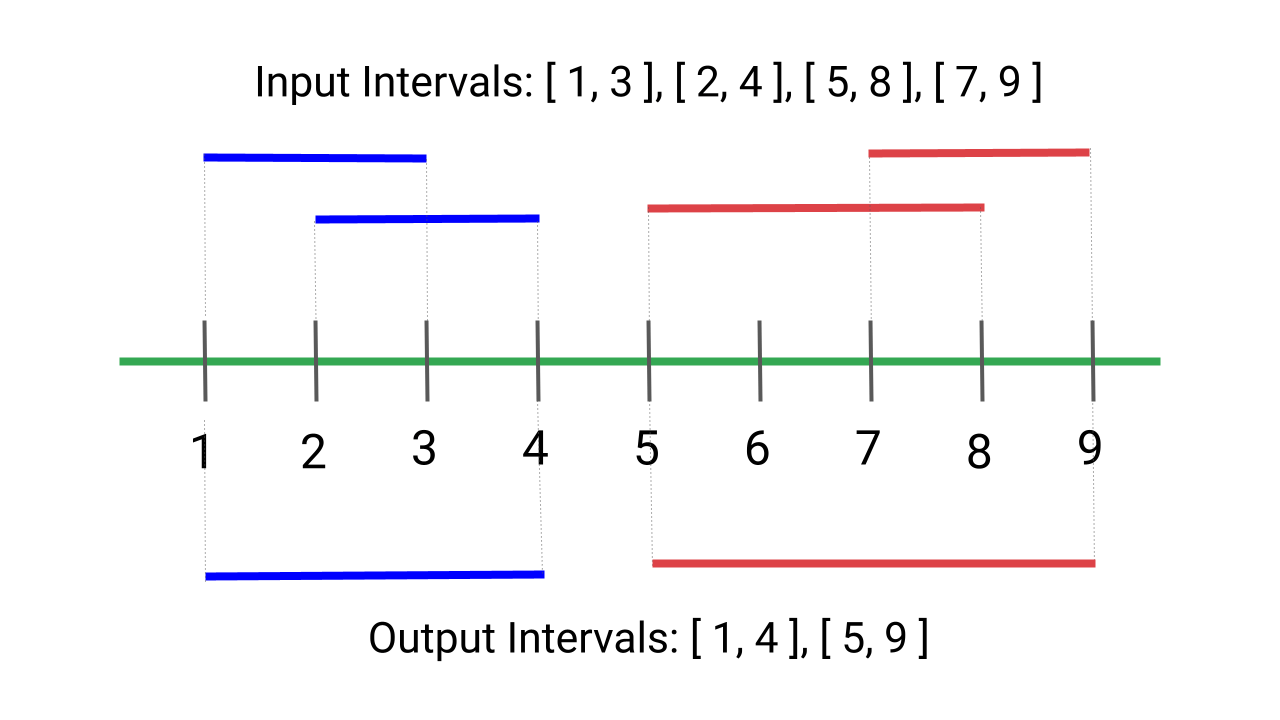Merge Overlapping Intervals

Given an array of intervals where the start and end points of each interval are provided, write a program to merge all overlapping intervals and return an array of non-overlapping intervals. In other words, the output should be an array of mutually exclusive intervals. Note: This is an excellent problem to learn problem solving using sorting.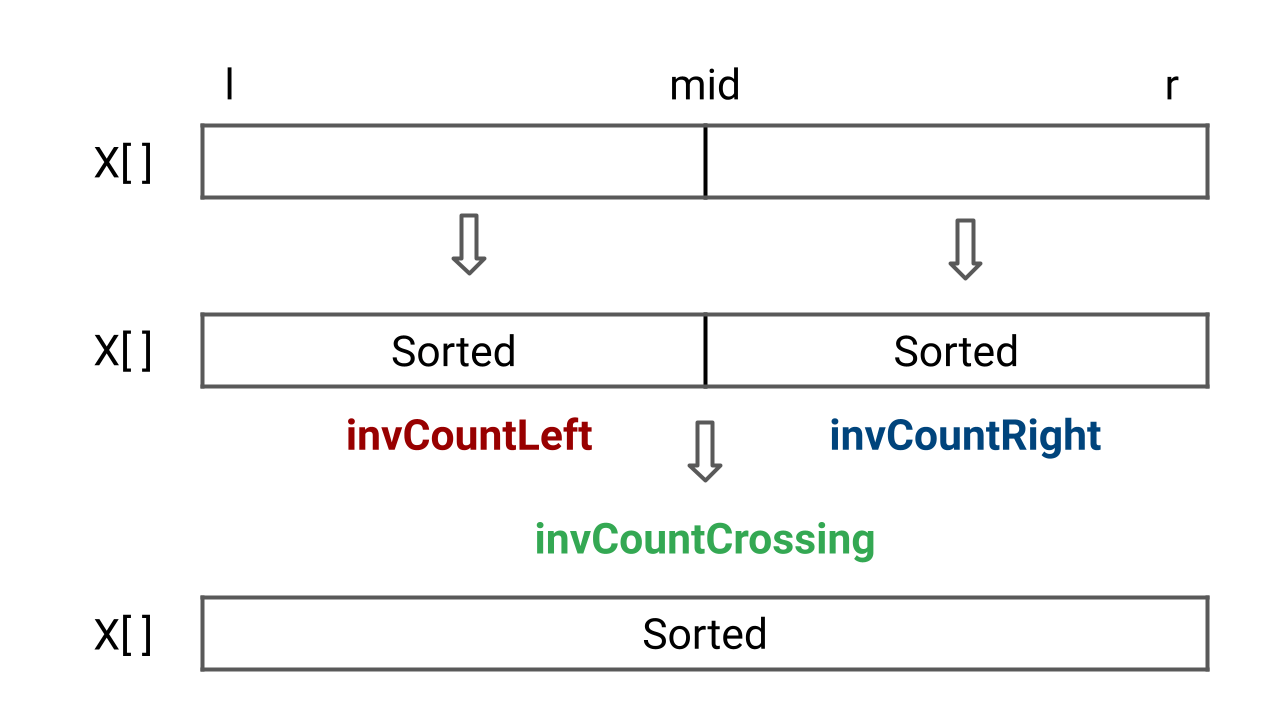Count Inversions of an Array

Given an array of n integers, write a program to find the total number of inversion counts. An inversion occurs when there are two elements in the array such that i < j and X[i] > X[j]. Here, the pair (i, j) is called an inversion of X[], and the inversion count represents the count of such inversions present in the array.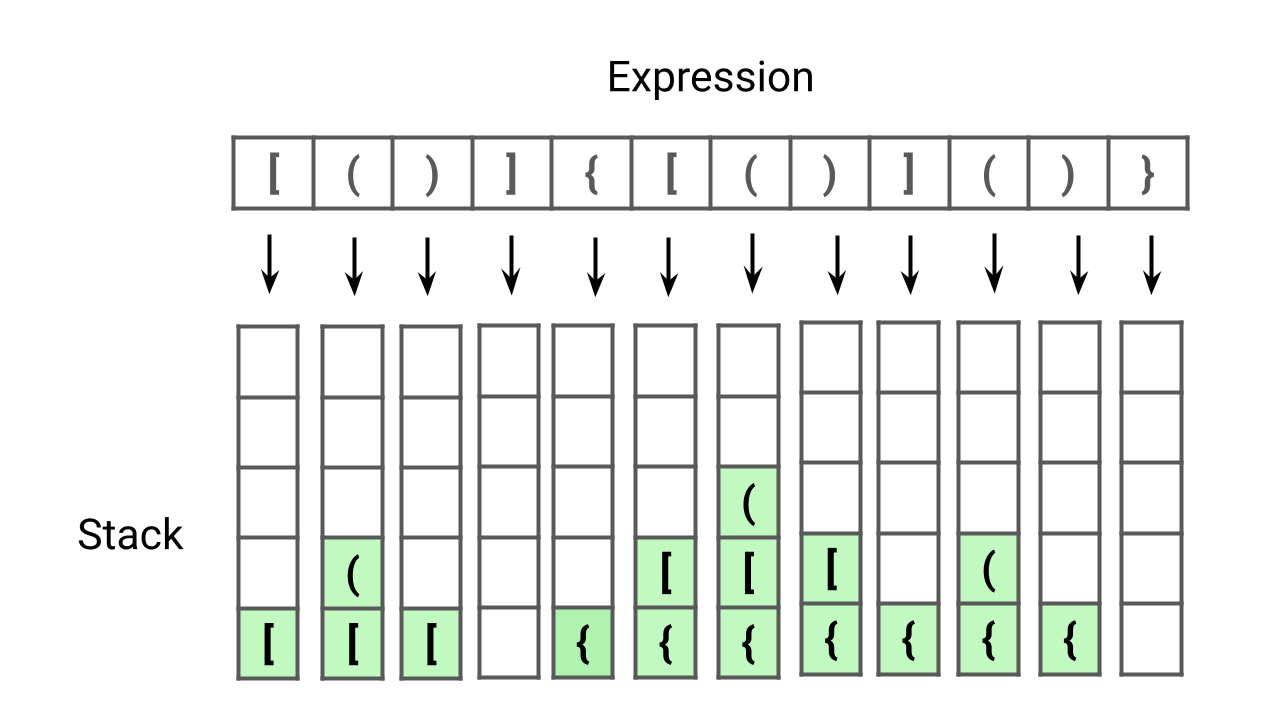Valid Parentheses Expression

Given an expression string containing opening and closing parentheses, write a program to check if the expression is balanced or not. An expression is considered balanced if each opening parenthesis is closed by the same type of closing parenthesis in the exact same order. This problem is excellent for learning problem-solving using stacks.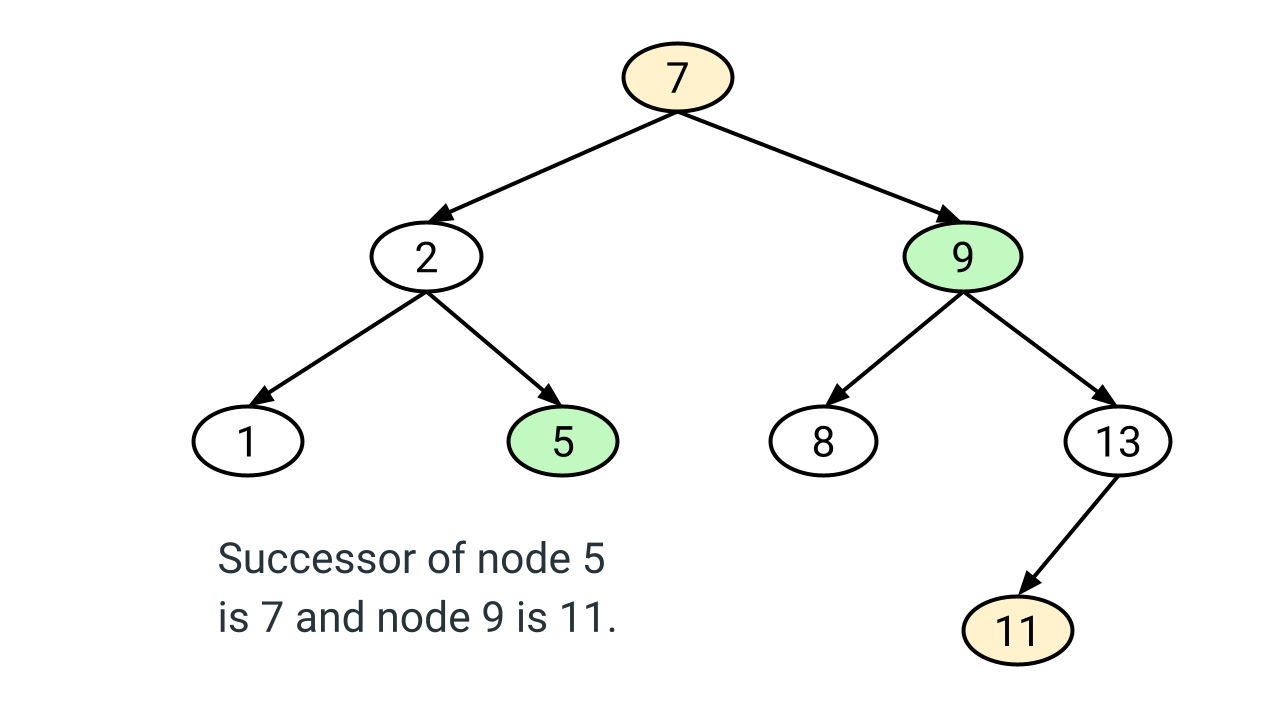Inorder Successor of a Node in Binary Search Tree (BST)

Given a node x and the root of a binary search tree, write a program to find the in-order successor of node x. The in-order successor of node x is a node that will be visited just after node x during an in-order traversal. In other words, the in-order successor of a node x is the node with the smallest key greater than x->key.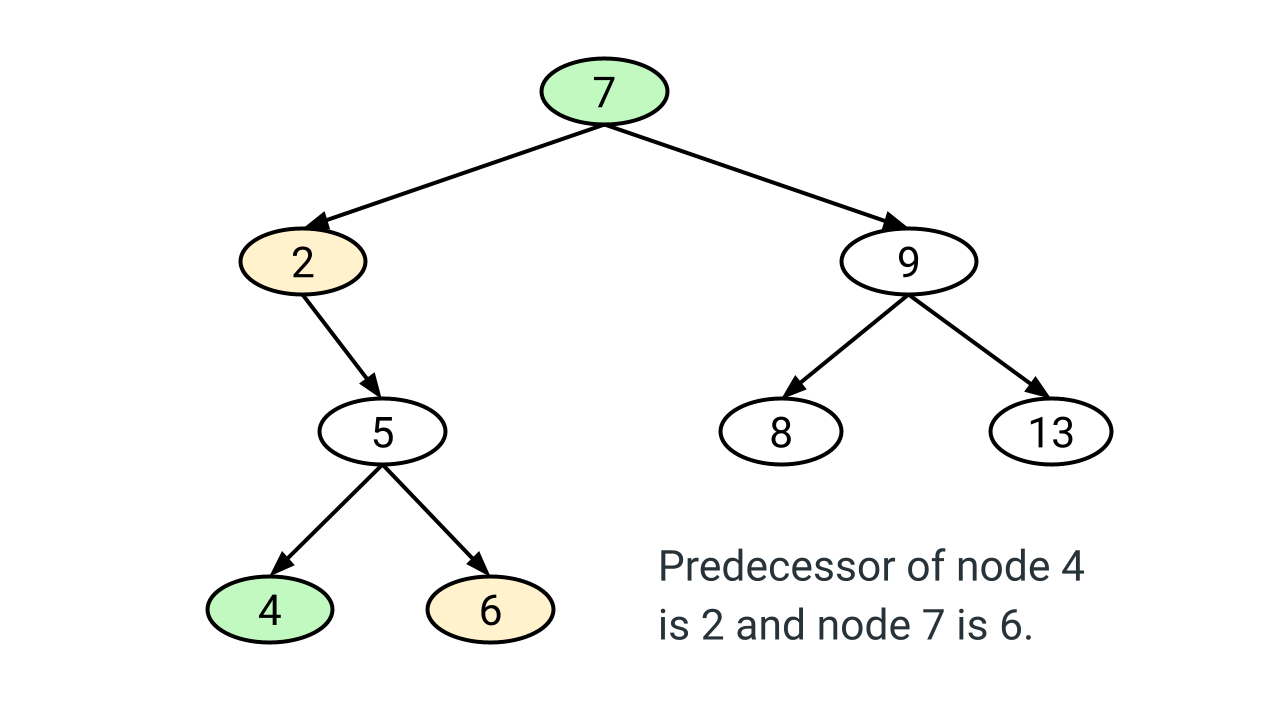Inorder Predecessor of a Node in Binary Search Tree (BST)

Write a program to find the in-order predecessor of a given node x in a binary search tree (BST). An in-order predecessor of node x is the node that will be visited just before node x in the in-order traversal. If the node x is visited first (leftmost node in BST) then the predecessor of node x is NULL.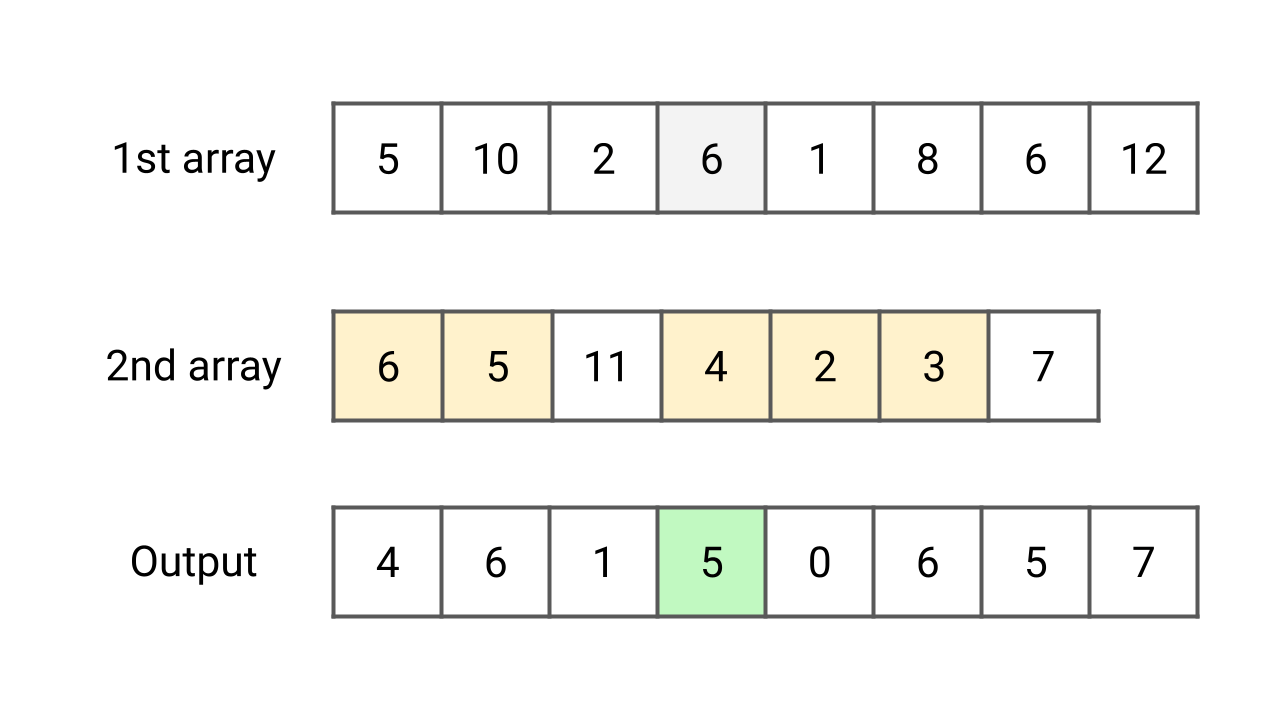Each value in 1st array count values less than or equal to in 2nd array

Given two unsorted arrays X[] and Y[] of size m and n. For each element in the first array X[] count the elements in the second array Y[] whose value is less than or equal to it. There can be duplicate elements in the arrays. Note: This is an excellent problem to learn problem solving using sorting and searching.Merge Sort Algorithm

Merge sort is one of the fastest comparison-based sorting algorithms, which works on the idea of a divide and conquer approach. The worst and best-case time complexity of the merge sort is O(nlogn), and the space complexity is O(n). It is also one of the best algorithms for sorting linked lists and learning the design and analysis of recursive algorithms.Binary Search Algorithm

Binary search is an efficient algorithm for searching a value in a sorted array using the divide and conquer idea. It compares the target value with the value at the mid-index and repeatedly reduces the search interval by half. The search continues until the value is found or the subarray size gets reduced to 0. The time complexity of the binary search is O(log n).Longest Consecutive Sequence

Given an array of n integers, find the length of the longest consecutive sequence. In other words, we need to find the length of the longest subsequence such that elements in the subsequence are consecutive integers. The consecutive numbers can be in any order. Note: This is an excellent problem to learn problem-solving using sorting and hash table.Longest Common Subsequence

Given two strings X[] and Y[] of sizes m and n, design an algorithm to find the length of the longest common subsequence (LCS). There can be many possible common subsequences of two strings, but we need to return the common subsequence of the longest length. Note that this is an excellent problem to learn dynamic programming.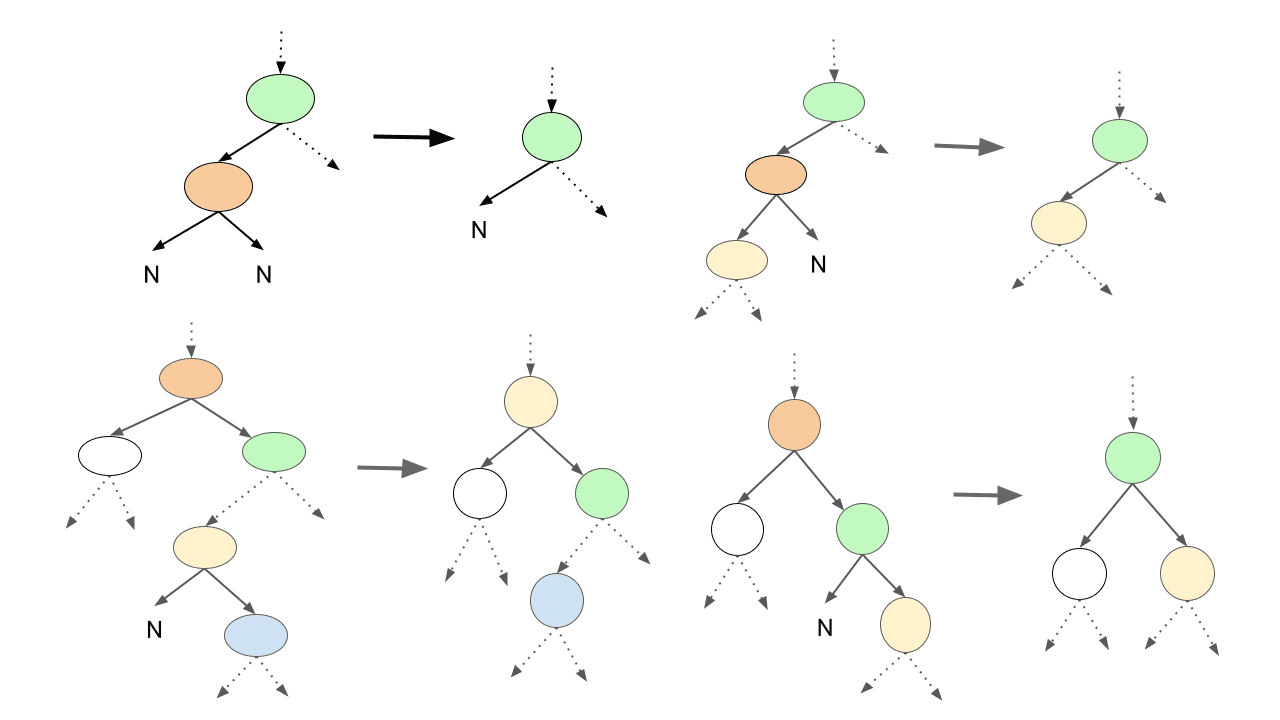Delete Node in a Binary Search Tree

Given a binary search tree and a key value. Write a program to delete the given key from the binary search tree and return the updated root node. This is an excellent problem to learn pointer manipulation in binary trees and problem-solving using both iterative and recursive approaches.Pastebin System Design

Pastebin is a online content hosting service where users can store and share content in the form of text or images over the internet by generating a unique URL so that anyone can access the content via that URL. Users can also update the content if they are logged in. This blog will focus on pastebin system design and discussion around various components.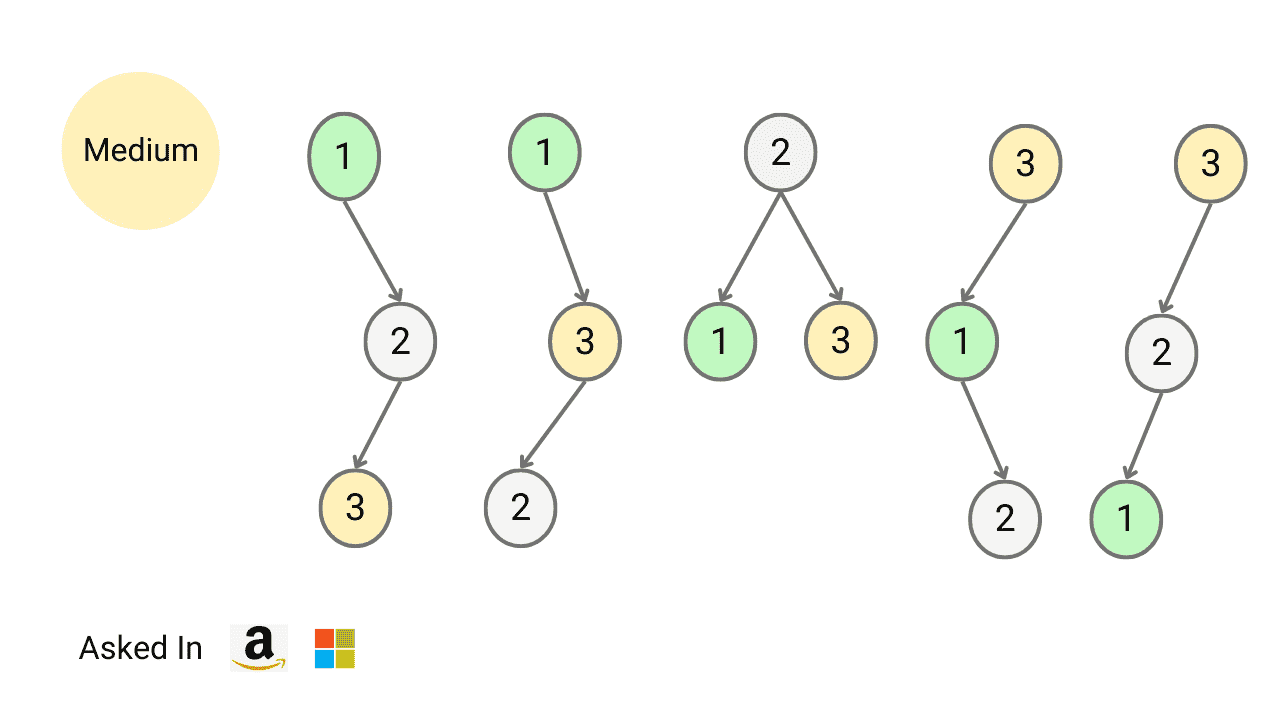Count Total Number of Unique Binary Search Trees with n Keys

Write a program to find the number of structurally unique binary search trees (BSTs) that have exactly n nodes, where each node has a unique integer key ranging from 1 to n. In other words, we need to determine the count of all possible BSTs that can be formed using n distinct keys.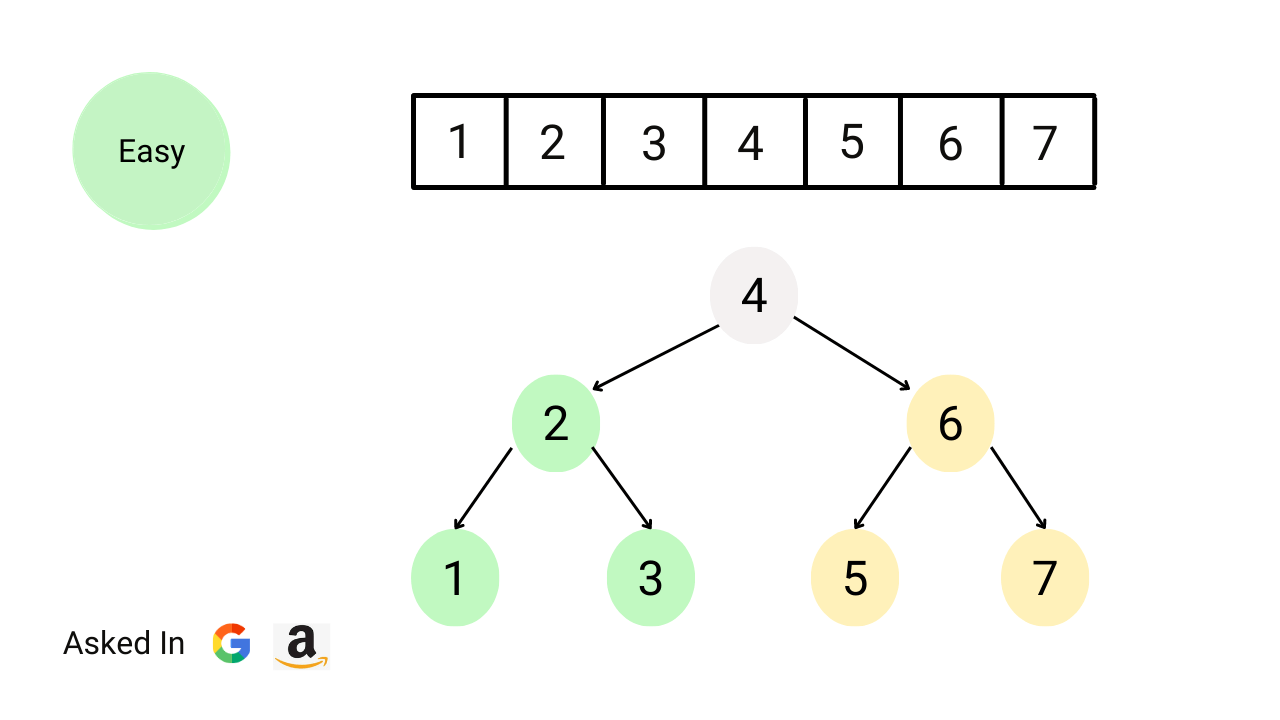Convert sorted array to balanced BST

Write a program to convert a sorted array of integers into a balanced binary search tree. Each node in the tree must follow the BST property, and the height of the left and right subtrees should be as close to equal as possible. Note: This is an excellent problem to learn problem-solving using the divide and conquer approach.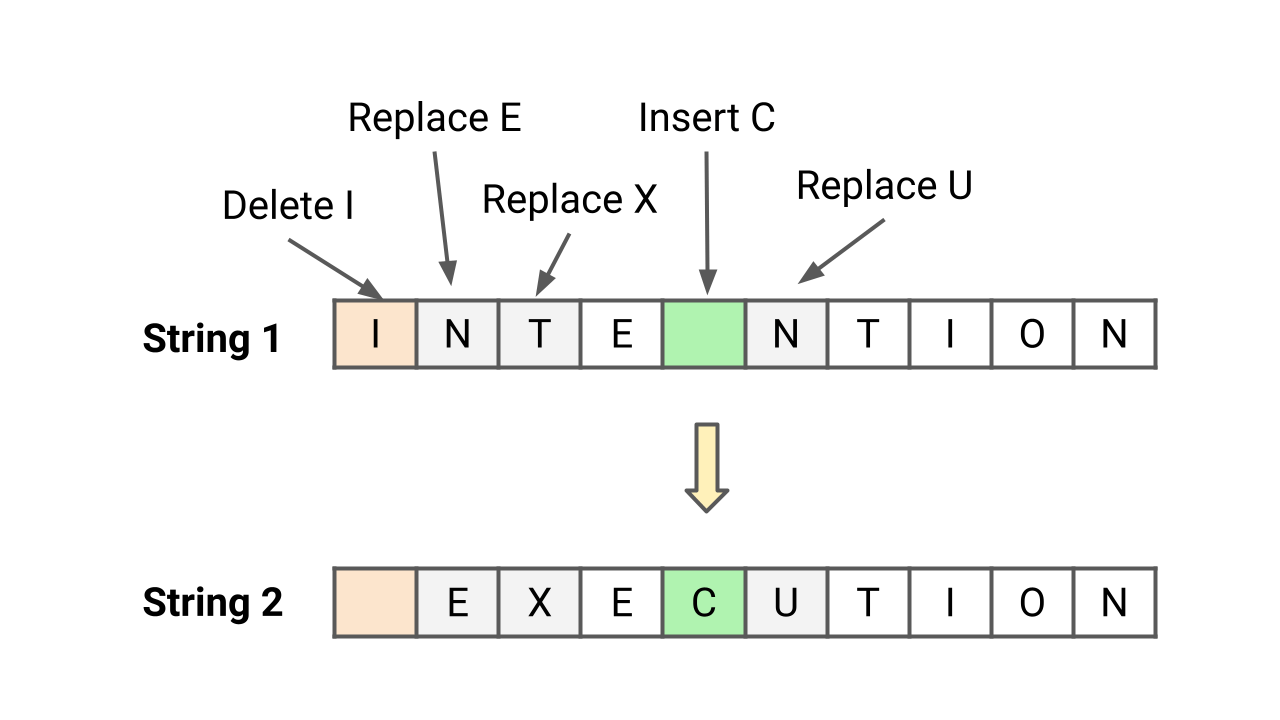Edit Distance (Levenshtein Distance) Problem

Write a program to find the minimum number of operations required to convert string X to string Y. You have the following three operations permitted on a string: 1) Insert a character 2) Delete a character 3) Replace a character. The edit distance between two strings is the minimum number of operations (insertions, deletions, or substitutions of characters) required to transform one string into the other.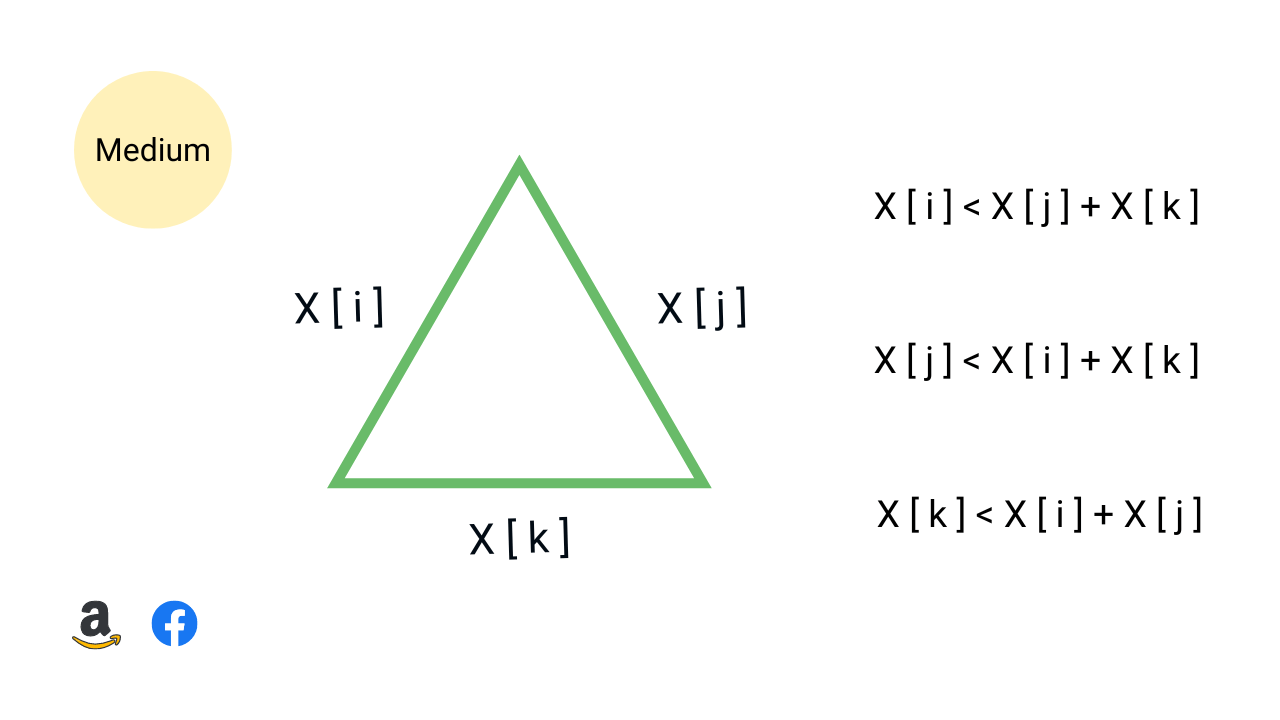Count the number of possible triangles

Write a program to count the number of possible triangles that can be formed with three elements from a given unsorted array of positive integers. The three elements, representing the lengths of the sides of a triangle, must satisfy the triangle inequality: the sum of any two sides must be greater than the third side.Find a fixed point in a given array

Write a program that returns a fixed point in the given sorted array of distinct integers. A fixed point is an index i such that X[i] = i. If a fixed point exists, the program should return the index of the fixed point. If no fixed point is found, the program should return -1. Note that the integers in the array can be both positive and negative.Merge Two Binary Trees

Given two binary trees, write a program to merge them into a single binary tree. We need to merge them such that if two nodes overlap then add them and put them into the new tree at the same position. Otherwise, put the non-NULL node in the new tree. Note: This is an excellent problem to learn problem solving using iterative and recursive preorder traversal.Counting Sort Algorithm

Counting sort is a stable sorting algorithm that works in O(n) time and space complexity when input are integers in the range 0 to k and k = O(n). Instead of comparison, counting sort uses array indexing to determine position of elements. For each element x, it counts values less than x and places x directly into its correct position in the sorted array.Detect Loop or Cycle in Linked List

Given the head of a linked list, write a program to find if linked list has a cycle or not. Return true if there is a cycle or loop in the linked list. Otherwise, return false. A linked list with cycle causes iteration to fail because the iteration will never reach the end. So, detecting a linked list loop is important before applying an iterative approach.Height (Maximum Depth) of a Binary Tree

Given a binary tree, write a program to find its height. In other words, we are given a binary tree and we need to calculate the maximum depth of the binary tree. The height or maximum depth of a binary tree is the total number of edges on the longest path from the root node to the leaf node. Note: This is an excellent problem to learn problem-solving using DFS and BFS traversal.Kth Largest Element in BST

Given the root of a BST and an integer k, write a program to find the kth largest value among all the nodes' values in the binary search tree. Note: This is an excellent problem to learn problem solving using recursive and iterative inorder traversal and data structure augmentation (storing extra information inside BST nodes for solving a problem).Implement Stack using Queues

Write a program to implement a stack using queues. We must use queue operations like enqueue, dequeue, front, size to implement stack operations like push, pop, and top. We have discussed three approaches: 1) Using two queues: O(n) pop and O(1) push 2) Using two queues: O(1) pop and O(n) push 3) Using one queue: O(1) pop and O(n) push.Implement Queue Using Stacks

Write a program to implement queue using stack. We should use stack operations like push, pop, top, size, and isEmpty for implementing queue operations like enqueue, dequeue, and front. In this blog, we have discussed two approaches for implementing queue using two stacks: 1) Dequeue O(1) and Enqueue O(n) 2) Enqueue O(1) and Dequeue O(1).Kth Smallest Element in an Array

Given an array and a positive integer k, write a program to find the kth smallest element in the array. This is an excellent problem to learn problem-solving using the max and min heap data structures. The quick-select approach (divide and conquer) is also worth exploring because it helps to optimize time complexity to O(n) on average.Climbing Stairs Problem

There is a staircase with n steps, and you can climb either 1 or 2 steps at a time. Write a program to count and return the number of unique ways to climb the nth stair. The order of steps taken matters. Note: Climbing stairs is an excellent problem to learn the dynamic programming approach and the application of the Fibonacci series in problem-solving.Minimum Coin Change Problem

Suppose we want to make a change for a given value K of cents, and we have an infinite supply of each of coin[ ] = [C​1​​, C​2​​, …, C​m​​] valued coins. Write a program to find the minimum number of coins required to make the change. Note: This is an excellent counting problem to learn problem solving using dynamic programming approach.Find Next Greater Element

Given an array, find the next greater element for every element in the array (NGE). The next greatest element for an element is the first largest element on the right side. For elements for which no next largest element exists, consider the next greater element as -1. We have discussed two stack-based solutions: 1) Traversing from left to right, 2) Traversing from right to left.Remove Duplicates from Sorted Array

Given a sorted array, write a program to remove duplicates from the array. We need to remove repeated elements so that there is a single occurrence of each element and return the length of the array containing unique elements. Note: This is an excellent problem to learn the fast and slow pointers approach. We have discussed two O(n) time in-place solutions.Row With Max 1s

Given a boolean 2D array, where each row is sorted. Find the row with the maximum number of 1s. This is an excellent matrix problem that can be solved in linear time complexity. The best part is — we are using the sorted order property and nested loops to improve the solution over the binary search approach.Find First and Last Position of Element in Sorted Array

Given an array of integers sorted in ascending order, the program should find the first and last occurrence of a given element. The goal is to design an algorithm with O(log n) time complexity. Note that this is an excellent question to learn problem-solving using binary search. Here, the binary search needs to be applied twice to solve this problem.Max Continuous Series of 1s

You are given an array of 1s and 0s and you are given an integer k which signifies the number of flips allowed. Write a program to find the position of zeros which when flipped will produce a maximum continuous series of 1s. Note: This is an excellent problem to learn problem solving using sliding window technique in O(n) time and O(1) space.Majority Element

You are given an array X[] of n elements, write a program to find majority element in an array. A majority element is an element that appears more than n/2 times, so there is at most one such element. Assume that array is non-empty and majority element always exists in the array. Note: This is an excellent problem to learn various approaches.Maximum Index

Given an array X[] of integers, find the maximum parity index. The parity index is the maximum index difference between two elements (X[i], X[j]) such that, j > i and X[j] > X[i]. In other words, we need to find maximum j - i such that j > i and X[j] > X[i]. Note: This is an excellent problem to learn problem solving using sorting and two pointers approachesMaximum Difference in an Array

Given an array A[] of n integers, find out the maximum difference between any two elements such that the larger element appears after the smaller element. In other words, we need to find max(A[j] - A[i]), where A[j] > A[i] and j > i. Note: This is an excellent problem to learn problem-solving using divide and conquer and a single loop.Find Triplets with Zero Sum

Given an array X[] of n distinct elements, write a program to find all the unique triplets in the array whose sum is equal to zero. For example, if triplets with zero sum in the array are (X[i], X[j], X[k]), then X[i] + X[j] + X[k] = 0. Hint: This is an excellent problem to learn problem-solving and optimization using hashing and two pointers approach.First Missing Positive

Given an array that includes both positive and negative numbers, write a program to find the first missing positive integer. This is one of the best problems for learning step-by-step time complexity optimization using various approaches. An in-place hashing solution uses the same input array to process values and generate output.Max Consecutive Ones

A binary array X[] is given where elements are either 0 or 1. Write a program to find the maximum consecutive ones. The subarray with max continuous 1's can be present anywhere, starting from some index i and ending at some index j. This is an excellent problem to learn problem solving using a sliding window approach and single loop.Longest Substring Without Repeating Characters

Given a string S, write a program to find the length of longest substring without repeating characters. The substring is a continuous subpart of the string and we need to return the largest substring which has all unique characters. Note: This is an excellent problem to learn problem solving and time complexity optimization using sliding window approach.Container With Most Water

Given an array of n non-negative integers height[n], where each represents a point at coordinate (i, height[i]). n vertical lines are drawn such that endpoints of line i is at (i, height[i]) and (i, 0). Find two lines, which together with x-axis form a container, such that container contains the most water. This is an excellent problem to learn two pointers approach.Trapping Rain Water

Given n non-negative integers representing an elevation map where the width of each bar is 1. Write a program to compute how much water it can trap after raining. This is a famous interview problem to learn time and space complexity optimization using various approaches. Two pointers approach provides an efficient solution using O(n) time and O(1) space.Sort an Array of 0s, 1s and 2s (Dutch National Flag Problem)

Given an array consisting of 0s, 1s, and 2s, write a program to sort this array of 0, 1, and 2 in ascending order. We need to sort the array in O(n) time complexity without using sorting algorithms or extra space. Note: This is a variation of the Dutch national flag problem and an excellent problem to learn problem solving using three pointers.Search in a row wise and column wise sorted matrix

You have given row-wise and column-wise sorted 2d matrix and integer k, write a program to search k in 2d matrix, i.e. find whether k is present or not. Each row is sorted from left to right, and the first integer of each row is greater than the last integer of the previous row. Note: This is an excellent problem to learn problem solving binary search in a 2d matrix.Find Maximum Element in a Bitonic Array

You are given an array of n integers that is first increasing and then decreasing. Write a program to find the maximum value in the array. Our goal should be to solve this problem using O(logn) time complexity. Note: This is an excellent problem to learn problem solving using binary search, where we modify binary search to get an efficient solution.Product of array except self

Given an array X[] of n integers, write a program to find product[] array such that product[i] is equal to product of all array elements except X[i]. We need to solve this problem without using division operations. Note: This is an excellent product array puzzle to learn time complexity optimization using prefix array and a single loop.Equilibrium Index of an Array

Write a program to find the equilibrium index of an array. An array's equilibrium index is an index such that the sum of elements at lower indexes equals the sum of elements at higher indexes. Note: This is an excellent coding question to learn time and space complexity optimization using a prefix array and a single loop using variables.Number of Buildings Facing Sun

Given an input array height[] which represents the heights of buildings, write a program to count the number of buildings facing the sunset. Assume that the heights of buildings are distinct. Insight: Here all buildings facing sunlight will be in increasing order. Note: This is an excellent problem to learn problem-solving using a single loop.FizzBuzz Problem

Given an integer n, write a program to return string representation of numbers from 1 to n. If number is divisible by 3, we store “Fizz”. If number is divisible by 5, we store “Buzz”. If number is divisible by both 3 and 5, we store “FizzBuzz”. Hint: This is an excellent puzzle to learn coding concepts like conditional statements, loops, etc.Write a program to find intersection of two sorted linked lists and return the head pointer of the new linked list. Here head pointers of both sorted linked lists are given as input and we shouldn’t do any changes in the structure to generate the output. Note: This is an excellent problem to learn problem-solving using recursion and two pointers in a linked list.Check whether two strings are anagram or not

Given two strings, str1 and str2, of size m and n, write a program to check whether two strings are an anagram of each other or not. A string str1 is an anagram of str2 if characters of str1 can be rearranged to form str2. Note: This is an excellent string problem to learn problem-solving and optimization using direct address table.Rotate Matrix by 90 Degrees

Given an n x n 2D matrix, write a program to rotate the matrix by 90 degrees in the anticlockwise direction. The program should rotate the matrix 90 degrees without using extra space. In other words, we have to perform the rotation by modifying the 2D matrix directly. Note that this is an excellent problem to learn problem-solving using loops and the transpose of a matrix.Spiral Traversal of Matrix

Given a 2-dimensional matrix, write a program to print matrix elements in spiral order. We can imagine spiral traversal as an ordered set of matrix segments with horizontal and vertical boundaries, where both boundaries are reduced by one at each step. Note: This is an excellent problem to learn problem-solving using iteration and recursion.Remove nth node from end of list

The head pointer of a linked list is given. Write a program to remove the Nth node from the end of the linked list and return its head. In other words, when the node is traversed from the end, we have to delete the Nth node from there. Note: This is an excellent problem for beginners to learn problem-solving using two pointers in a linked list.A head pointer of a singly linked list is given, write a program to reverse linked list and return the head pointer of the reversed list. We need to reverse the list by changing the links between nodes. Note: This is an excellent question to learn problem-solving using both iteration (Three-pointers) and recursion (Decrease and conquer approach).Given a singly linked list, write a program to find the middle element of the linked list. We need to return the second middle node if the node count is even. The goal should be to use a single loop and O(1) extra space to find the middle element. Note: This is an excellent problem to learn problem-solving using fast and slow pointers in the linked list.Largest Subarray With 0 Sum

Given an array X[] of n integers, write a program to find the length of largest continuous subarray with zero sum. The subarray length starting from index i and ending at index j will be j - i + 1. So in other words, for all j > i, find max (j - i + 1) among all subarrays with zero sum.. Note: This is an excellent question to learn problem-solving using hash table.Wave Array Problem

Given an unsorted array of n integers, write a program to sort array into a wave array. There can be many possible waveforms, but we need to return any one of them. An array A[] is sorted in wave arrangement if A >= A <= A >= A <= A >= ....and so on. Note: This is an excellent problem to learn problem-solving using a single loop.Find Minimum in Rotated Sorted Array

A sorted and rotated array of size n is given, write a program to find minimum element in rotated sorted array. Rotation by k times means that the first k sorted elements of the array will move to the last k positions, and the last n - k sorted elements will move to the first n - k positions. Note: We don’t know how many times array is rotated in the given problem.Check if two arrays are equal or not

Given two integer arrays X[] and Y[], write a program to check if arrays are equal or not. Two arrays are equal if they have the same elements in any order. If there are repeated elements, then counts of repeated elements must also be the same for both arrays. Note: This is an excellent problem to learn problem solving using a hash table.Roman to Integer

Given a Roman number, write a program to convert Roman number to a corresponding integer value. What are Roman numbers? Roman numbers are the symbols used in a system of numerical notation based on the ancient Roman system. The symbols are I, V, X, L, C, D, and M, which represent integer values respectively for 1, 5, 10, 50, 100, 500, and 1,000.Given an integer array X[] of size n, write a program to find all the leaders present in the array X[]. An array element is a leader if it is strictly greater than all elements to its right side. So the largest and last element of an array is a leader by default. Note: This is an excellent problem to learn problem-solving using a single loop and variables.Valid Mountain Array

Given an array X[] of n integers, return true if it is a valid mountain array. The array X[] is a mountain array if and only if n >= 3 and there exists some i with 0 < i < n - 1 such that: X < X <...X[i-1] < X[i] and X[i] > X[i+1] > ...> X[n - 1]. In other words, we call the array mountain array when the array is strictly increasing and then strictly decreasing.Validate Binary Search Tree (BST)

Given the root of a binary tree, write a program to check whether tree is a valid binary search tree (BST) or not. To check valid bst, we verify bst property at each node: All node values in the left subtree are less than node’s value, all node values in the right subtree are greater than node’s value, and both subtrees are also binary search trees.Left View of Binary Tree

Given a root node of a binary tree, write a program to find the left view of the given binary tree. The left view of a binary tree is a group of nodes visible when viewed from the left side. In other words, the nodes in the left view will be the first node of each level. This is an excellent problem to learn problem-solving using DFS and BFS traversal.Minimum Depth of Binary Tree

Given a binary tree, find its minimum depth. The min depth of a binary tree is the number of nodes along the shortest path from root node down to the nearest leaf node. The path has to end on a leaf node. Note: An excellent problem to understand efficient problem solving using breadth-first search (BFS) when the solution node is nearest to the root node.